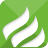Matplotlib 教程

介绍

Matplotlib 可能是 Python 2D-绘图领域使用最广泛的套件。它能让使用者很轻松地将数据图形化，并且提供多样化的输出格式。这里将会探索 matplotlib 的常见用法。

IPython 以及 pylab 模式

IPython 是 Python 的一个增强版本。它在下列方面有所增强：命名输入输出、使用系统命令（shell commands）、排错（debug）能力。我们在命令行终端给 IPython 加上参数 -pylab （0.12 以后的版本是 --pylab）之后，就可以像 Matlab 或者 Mathematica 那样以交互的方式绘图。

pylab

pylab 是 matplotlib 面向对象绘图库的一个接口。它的语法和 Matlab 十分相近。也就是说，它主要的绘图命令和 Matlab 对应的命令有相似的参数。

• figures：存放实例代码生成的图片

• scripts：存放实例代码

初级绘制

`from pylab import *X = np.linspace(-np.pi, np.pi, 256,endpoint=True)C,S = np.cos(X), np.sin(X)`

X 是一个 numpy 数组，包含了从 −π 到 +π 等间隔的 256 个值。C 和 S 则分别是这 256 个值对应的余弦和正弦函数值组成的 numpy 数组。

`python exercise_1.py`

使用默认配置[源码文件]

Matplotlib 的默认配置都允许用户自定义。你可以调整大多数的默认配置：图片大小和分辨率（dpi）、线宽、颜色、风格、坐标轴、坐标轴以及网格的属性、文字与字体属性等。不过，matplotlib 的默认配置在大多数情况下已经做得足够好，你可能只在很少的情况下才会想更改这些默认配置。

```import numpy as npimport matplotlib.pyplot as plt

X = np.linspace(-np.pi, np.pi, 256, endpoint=True)C,S = np.cos(X), np.sin(X)plt.plot(X,C)plt.plot(X,S)plt.show()```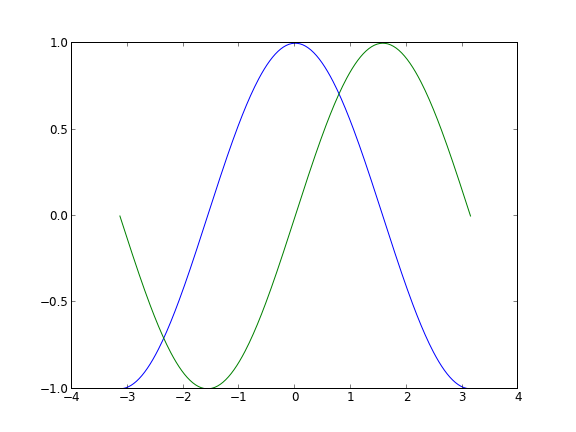默认配置的具体内容[源码文件]

`# 导入 matplotlib 的所有内容（nympy 可以用 np 这个名字来使用）from pylab import *# 创建一个 8 * 6 点（point）的图，并设置分辨率为 80figure(figsize=(8,6), dpi=80)# 创建一个新的 1 * 1 的子图，接下来的图样绘制在其中的第 1 块（也是唯一的一块）subplot(1,1,1)X = np.linspace(-np.pi, np.pi, 256,endpoint=True)C,S = np.cos(X), np.sin(X)# 绘制余弦曲线，使用蓝色的、连续的、宽度为 1 （像素）的线条plot(X, C, color="blue", linewidth=1.0, linestyle="-")# 绘制正弦曲线，使用绿色的、连续的、宽度为 1 （像素）的线条plot(X, S, color="green", linewidth=1.0, linestyle="-")# 设置横轴的上下限xlim(-4.0,4.0)# 设置横轴记号xticks(np.linspace(-4,4,9,endpoint=True))# 设置纵轴的上下限ylim(-1.0,1.0)# 设置纵轴记号yticks(np.linspace(-1,1,5,endpoint=True))# 以分辨率 72 来保存图片# savefig("exercice_2.png",dpi=72)# 在屏幕上显示show()`改变线条的颜色和粗细[源码文件]

`...figure(figsize=(10,6), dpi=80)plot(X, C, color="blue", linewidth=2.5, linestyle="-")plot(X, S, color="red",  linewidth=2.5, linestyle="-")...`设置图片边界[源码文件]

... xlim(X.min()*1.1, X.max()*1.1) ylim(C.min()*1.1, C.max()*1.1) ...

`xmin ,xmax = X.min(), X.max()ymin, ymax = Y.min(), Y.max()dx = (xmax - xmin) * 0.2dy = (ymax - ymin) * 0.2xlim(xmin - dx, xmax + dx)ylim(ymin - dy, ymax + dy)`设置记号[源码文件]

...

`xticks( [-np.pi, -np.pi/2, 0, np.pi/2, np.pi])yticks([-1, 0, +1])...`设置记号的标签[源码文件]

`...xticks([-np.pi, -np.pi/2, 0, np.pi/2, np.pi],       [r'\$-pi\$', r'\$-pi/2\$', r'\$0\$', r'\$+pi/2\$', r'\$+pi\$'])yticks([-1, 0, +1],       [r'\$-1\$', r'\$0\$', r'\$+1\$'])...`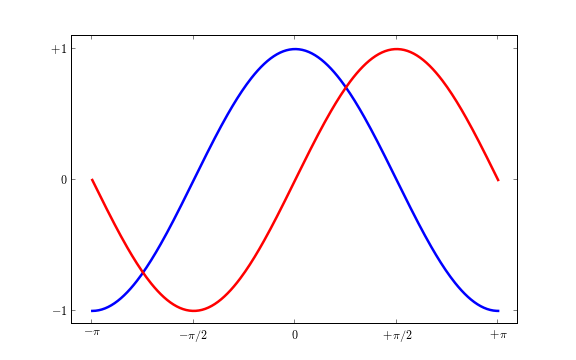移动脊柱[源码文件]

`...ax = gca()ax.spines['right'].set_color('none')ax.spines['top'].set_color('none')ax.xaxis.set_ticks_position('bottom')ax.spines['bottom'].set_position(('data',0))ax.yaxis.set_ticks_position('left')ax.spines['left'].set_position(('data',0))...`添加图例[源码文件]

`...plot(X, C, color="blue", linewidth=2.5, linestyle="-", label="cosine")plot(X, S, color="red",  linewidth=2.5, linestyle="-", label="sine")legend(loc='upper left')...`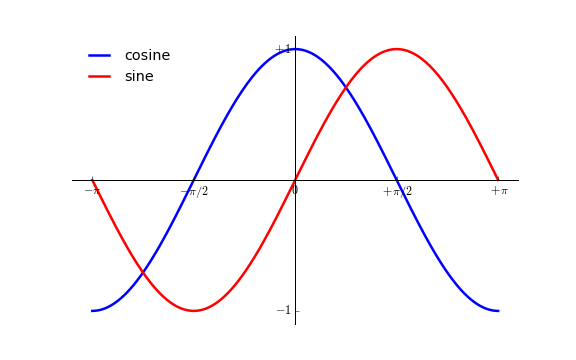给一些特殊点做注释[源码文件]

```...t = 2*np.pi/3plot([t,t],[0,np.cos(t)], color ='blue', linewidth=2.5, linestyle="--")scatter([t,],[np.cos(t),], 50, color ='blue')annotate(r'\$sin(frac{2pi}{3})=frac{sqrt{3}}{2}\$',
xy=(t, np.sin(t)), xycoords='data',
xytext=(+10, +30), textcoords='offset points', fontsize=16,
arrowprops=dict(arrowstyle="->", connectionstyle="arc3,rad=.2"))plot([t,t],[0,np.sin(t)], color ='red', linewidth=2.5, linestyle="--")scatter([t,],[np.sin(t),], 50, color ='red')annotate(r'\$cos(frac{2pi}{3})=-frac{1}{2}\$',
xy=(t, np.cos(t)), xycoords='data',
xytext=(-90, -50), textcoords='offset points', fontsize=16,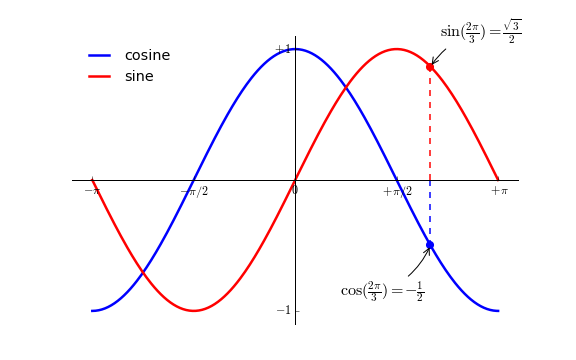精益求精[源码文件]

```...for label in ax.get_xticklabels() + ax.get_yticklabels():
label.set_fontsize(16)
label.set_bbox(dict(facecolor='white', edgecolor='None', alpha=0.65 ))...```图像

num 1 图像的数量
figsize figure.figsize 图像的长和宽（英寸）
dpi figure.dpi 分辨率（点/英寸）
facecolor figure.facecolor 绘图区域的背景颜色
edgecolor figure.edgecolor 绘图区域边缘的颜色
frameon True 是否绘制图像边缘

1. 不传递参数：关闭当前窗口；

2. 传递窗口编号或窗口实例（instance）作为参数：关闭指定的窗口；

3. `all`：关闭所有窗口。

子图

[源码文件][源码文件]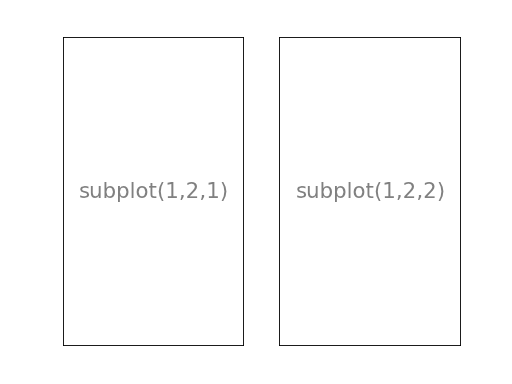[源码文件][源码文件]坐标轴

[源码文件]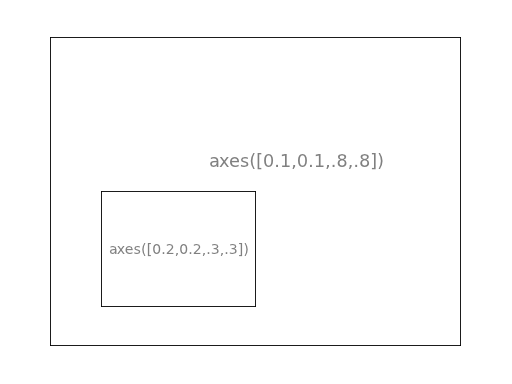[源码文件]Tick Locators

NullLocator No ticks.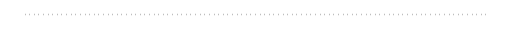IndexLocator Place a tick on every multiple of some base number of points plotted.FixedLocator Tick locations are fixed.LinearLocator Determine the tick locations.MultipleLocator Set a tick on every integer that is multiple of some base.AutoLocator Select no more than n intervals at nice locations.LogLocator Determine the tick locations for log axes.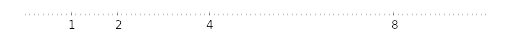普通图[源码文件]

`from pylab import *n = 256X = np.linspace(-np.pi,np.pi,n,endpoint=True)Y = np.sin(2*X)plot (X, Y+1, color='blue', alpha=1.00)plot (X, Y-1, color='blue', alpha=1.00)show()`散点图[源码文件]

`from pylab import *n = 1024X = np.random.normal(0,1,n)Y = np.random.normal(0,1,n)scatter(X,Y)show()`条形图[源码文件]

```from pylab import *n = 12X = np.arange(n)Y1 = (1-X/float(n)) * np.random.uniform(0.5,1.0,n)Y2 = (1-X/float(n)) * np.random.uniform(0.5,1.0,n)bar(X, +Y1, facecolor='#9999ff', edgecolor='white')bar(X, -Y2, facecolor='#ff9999', edgecolor='white')for x,y in zip(X,Y1):
text(x+0.4, y+0.05, '%.2f' % y, ha='center', va= 'bottom')ylim(-1.25,+1.25)show()```等高线图[源码文件]

`from pylab import *def f(x,y): return (1-x/2+x**5+y**3)*np.exp(-x**2-y**2)n = 256x = np.linspace(-3,3,n)y = np.linspace(-3,3,n)X,Y = np.meshgrid(x,y)contourf(X, Y, f(X,Y), 8, alpha=.75, cmap='jet')C = contour(X, Y, f(X,Y), 8, colors='black', linewidth=.5)show()`灰度图（Imshow）[源码文件]

`from pylab import *def f(x,y): return (1-x/2+x**5+y**3)*np.exp(-x**2-y**2)n = 10x = np.linspace(-3,3,4*n)y = np.linspace(-3,3,3*n)X,Y = np.meshgrid(x,y)imshow(f(X,Y)), show()`饼状图[源码文件]

`from pylab import *n = 20Z = np.random.uniform(0,1,n)pie(Z), show()`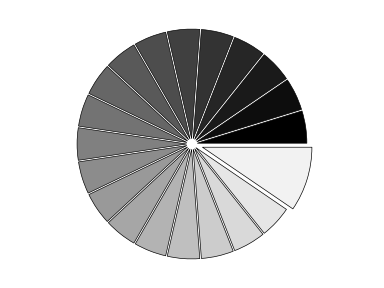量场图（Quiver Plots）[源码文件]

`from pylab import *n = 8X,Y = np.mgrid[0:n,0:n]quiver(X,Y), show()`网格[源码文件]

`from pylab import *axes = gca()axes.set_xlim(0,4)axes.set_ylim(0,3)axes.set_xticklabels([])axes.set_yticklabels([])show()`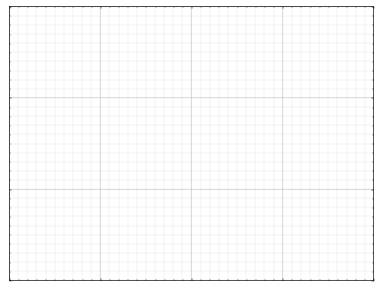多重网格[源码文件]

`from pylab import *subplot(2,2,1)subplot(2,2,3)subplot(2,2,4)show()`极轴图[源码文件]

```from pylab import *axes([0,0,1,1])N = 20theta = np.arange(0.0, 2*np.pi, 2*np.pi/N)radii = 10*np.random.rand(N)width = np.pi/4*np.random.rand(N)bars = bar(theta, radii, width=width, bottom=0.0)for r,bar in zip(radii, bars):
bar.set_facecolor( cm.jet(r/10.))
bar.set_alpha(0.5)show()```3D 图[源码文件]

`from pylab import *from mpl_toolkits.mplot3d import Axes3Dfig = figure()ax = Axes3D(fig)X = np.arange(-4, 4, 0.25)Y = np.arange(-4, 4, 0.25)X, Y = np.meshgrid(X, Y)R = np.sqrt(X**2 + Y**2)Z = np.sin(R)ax.plot_surface(X, Y, Z, rstride=1, cstride=1, cmap='hot')show()`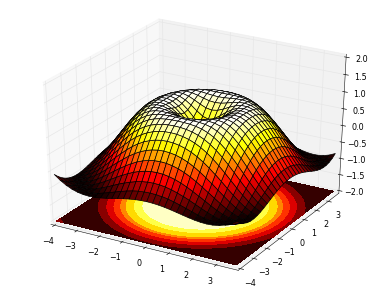手稿图[源码文件]

```import numpy as npimport matplotlib.pyplot as plt

eqs = []eqs.append((r"\$W^{3beta}_{delta_1 rho_1 sigma_2} = U^{3beta}_{delta_1 rho_1} + frac{1}{8 pi 2} int^{alpha_2}_{alpha_2} d alpha^prime_2 left[frac{ U^{2beta}_{delta_1 rho_1} - alpha^prime_2U^{1beta}_{rho_1 sigma_2} }{U^{0beta}_{rho_1 sigma_2}}right]\$"))eqs.append((r"\$frac{drho}{d t} + rho vec{v}cdotnablavec{v} = -nabla p + munabla^2 vec{v} + rho vec{g}\$"))eqs.append((r"\$int_{-infty}^infty e^{-x^2}dx=sqrt{pi}\$"))eqs.append((r"\$E = mc^2 = sqrt{{m_0}^2c^4 + p^2c^2}\$"))eqs.append((r"\$F_G = Gfrac{m_1m_2}{r^2}\$"))plt.axes([0.025,0.025,0.95,0.95])for i in range(24):
index = np.random.randint(0,len(eqs))
eq = eqs[index]
size = np.random.uniform(12,32)
x,y = np.random.uniform(0,1,2)
alpha = np.random.uniform(0.25,.75)
plt.text(x, y, eq, ha='center', va='center', color="#11557c", alpha=alpha,
transform=plt.gca().transAxes, fontsize=size, clip_on=True)plt.xticks([]), plt.yticks([])# savefig('../figures/text_ex.png',dpi=48)plt.show()```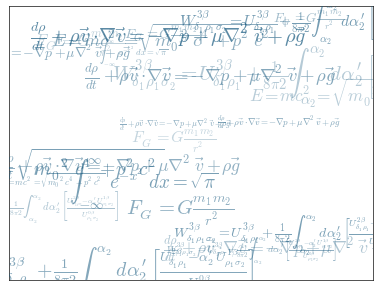更多参考

Matplotlib 文档

Numpy 文档

0% (0)
0% (0)
0.060735s